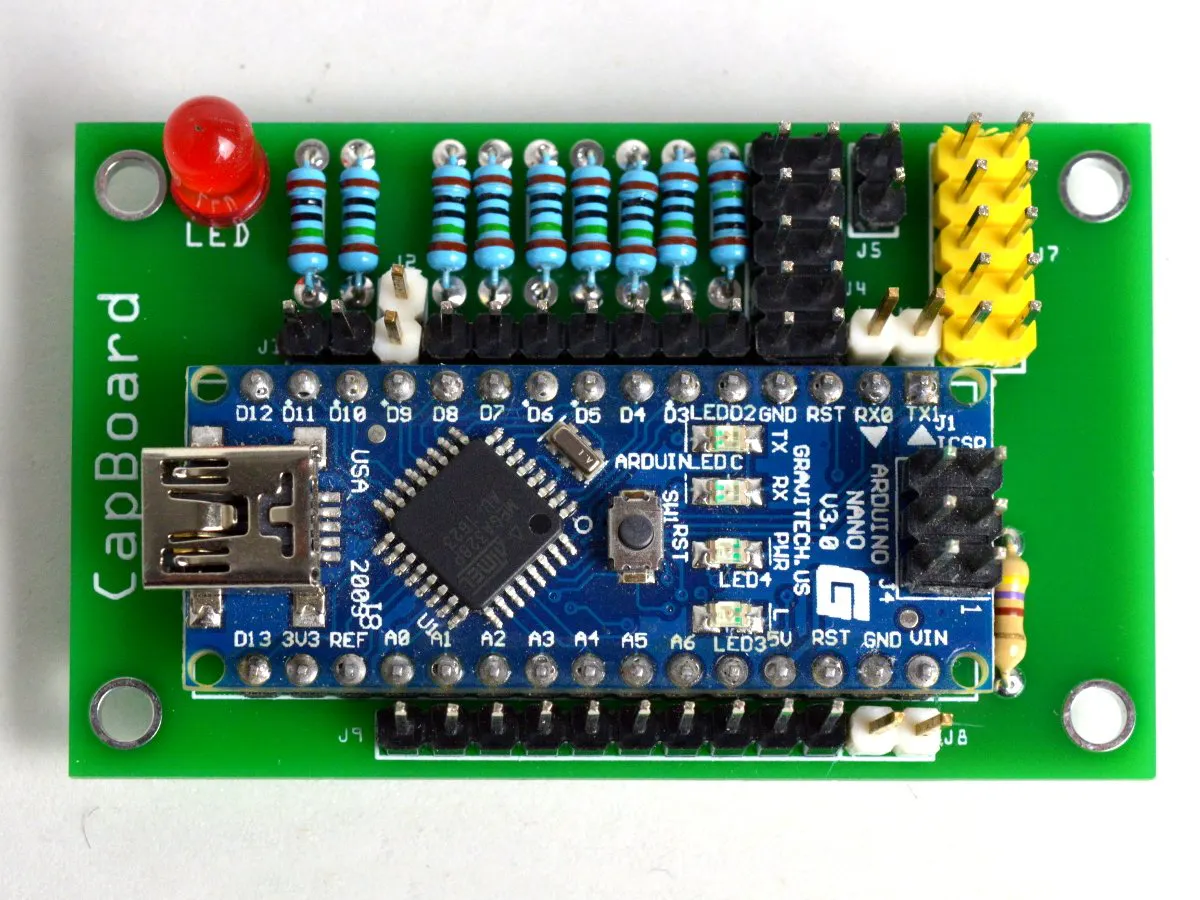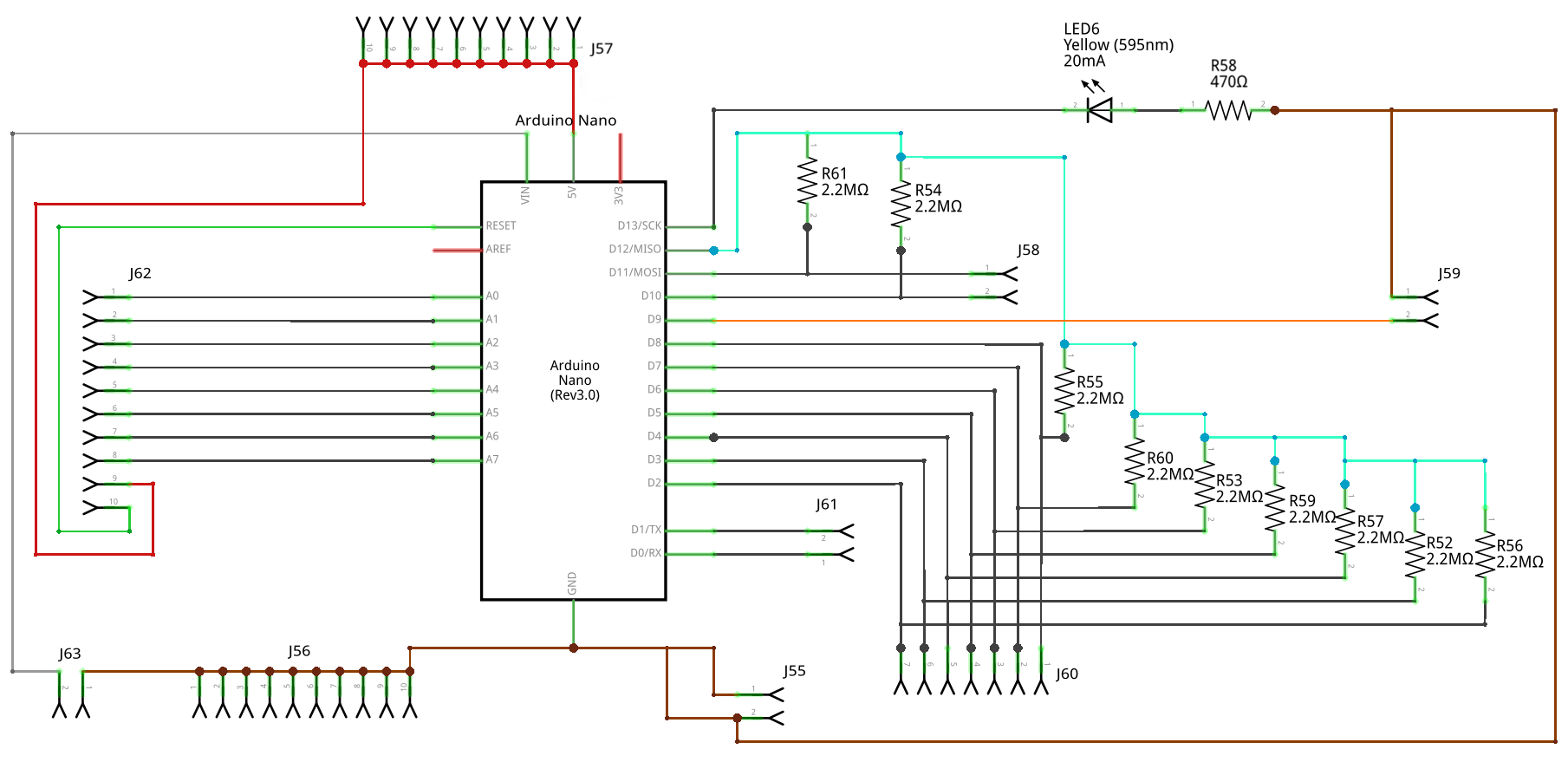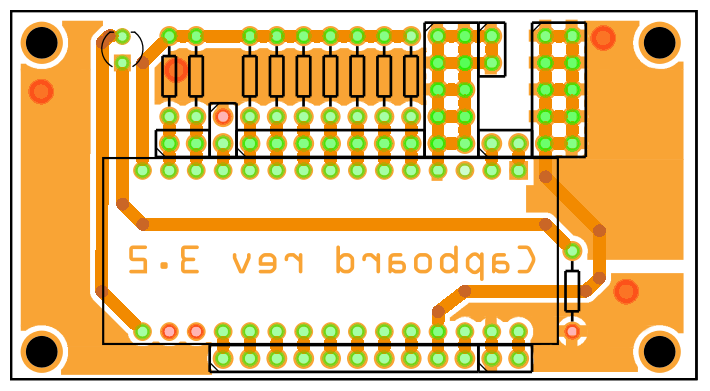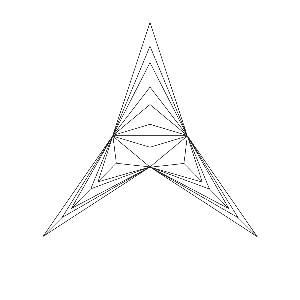Capacitive Board (CapBoard) is a device based on Arduino Nano for turning conductive objects into a capacitive sensor to detect the touch.

• 2,160 views
• 1 respect

## Components and supplies

The story

The board makes it possible to connect up to nine different conductive objects; also, it has eight analog inputs that can be used to connect potentiometers or other types of sensors.

If connected to a laptop, the board sends the data relating the state of the sensors via the serial connection. Currently, CapBoard has been interfaced with pure data and processing through a special Arduino sketch (available soon on this page).

### With CapBoard:

You can try to invent new ways to interact with your laptop.

The board is equipped with an LED connected to the digital port number 12, while port 13 is free and can be used to connect a speaker or an audio cable to generate sounds through the Arduino tone() function.

Here the first prototype of CapBoard.

### Programming CapBoard:

You can create an 8-bit synth controlled by touching the objects connect to the card. Obviously, it can also be connected to any other environment or software capable of receiving data via a serial connection.

Capacitive sensors to be connected to CapBoard can be made with aluminum foil, as those used for the prototype CapPad, or you can use any other metal objects as shown in the image.

This video shows an interactive sound installation in which CapBoard was used to make sensitive four tuff blocks. The installation has been set up for the exhibition Attraverso la città: La strada del Sole at San Nicola dei Greci in Matera from December 10, 2016 to February 12, 2017.

T

To program the CapBoard, you need to use the Arduino CapacitiveSensor library. For more information, refer to the official project page.

## Code

##### CapBoard-Test1-CapSensC/C++
How to create a capacitive sensor with CapBoard
```/*
Capacitive Sensor Test for CapBoard
Turns on and off a LED connected to the port defined by the variable LedPin
according to the state of a capacitive sensor connected to the port defined
by the variable SensorPin.

To use this sketch you need to install the CapacitiveSensor library.
http://playground.arduino.cc/Main/CapacitiveSensor?from=Main.CapSense

This example code is in the public domain.

Artis Lab - 2016
www.artislab.it
info@artislab.it
*/

#include <CapacitiveSensor.h>
boolean isTriggered1=false;
int Threshold=10000; //Change this value to calibrate the sensor
//Use the serial monitor to find the value provided by your sensor
int Samples=30;
int LedPin=13;
int SensorPin=2;
int Emitter=12;
CapacitiveSensor CapSensor = CapacitiveSensor(Emitter,SensorPin);

void setup(){
pinMode(LedPin,OUTPUT);
//uncomment the next line if you want to enable the serial communication with the laptop
Serial.begin(9600);
//CapSensor.set_CS_AutocaL_Millis(10000);
}

void loop(){

long total1=CapSensor.capacitiveSensor(Samples);
//uncomment the next line if you want to send the sensor data to the laptop over the serial communication
//Comment this line if you want to send the sensor state over the serial with Serial.write!!!
Serial.println(total1);
if(total1>Threshold)
{
if(!isTriggered1)
{
digitalWrite(LedPin,HIGH);
//Uncomment the following line to send data over the serial
//Serial.write(1);   //Sensor State
//Serial.write(206); //Sensor ID
isTriggered1=true;
//Uncomment the following line to print the state of the sensor
//Don't use it togheter with Serial.write!!!
//Serial.println("CapSens-On");
}
} else
{
if(isTriggered1)
{
digitalWrite(LedPin,LOW);
//Uncomment the following line to send data over the serial
//Serial.write(0);   //Sensor State
//Serial.write(206); //Sensor ID
isTriggered1=false;
//Uncomment the following line to print the state of the sensor
//Don't use it togheter with Serial.write!!!
//Serial.println("CapSens-Off");
}
}
}
```
##### CapBoard Nine Capacitive SensorsC/C++
How to create nine capacitive sensors with CapBoard
```#include <CapacitiveSensor.h>
CapacitiveSensor cs122 = CapacitiveSensor(12,2);
CapacitiveSensor cs123 = CapacitiveSensor(12,3);
CapacitiveSensor cs124 = CapacitiveSensor(12,4);
CapacitiveSensor cs125 = CapacitiveSensor(12,5);
CapacitiveSensor cs126 = CapacitiveSensor(12,6);
CapacitiveSensor cs127 = CapacitiveSensor(12,7);
CapacitiveSensor cs128 = CapacitiveSensor(12,8);
CapacitiveSensor cs1210 = CapacitiveSensor(12,10);
CapacitiveSensor cs1211 = CapacitiveSensor(12,11);
boolean isTriggered1=false;
boolean isTriggered2=false;
boolean isTriggered3=false;
boolean isTriggered4=false;
boolean isTriggered5=false;
boolean isTriggered6=false;
boolean isTriggered7=false;
boolean isTriggered8=false;
boolean isTriggered9=false;
int Soglia=2400;
int MaxSensorValue=80000;
int Samples=40;
int LedPin=13;
int Filter1=0;
int Filter2=0;
int Filter3=0;

int i = 0;
int lastValue0=0;
void setup(){
pinMode(LedPin,OUTPUT);
//cs23.set_CS_AutocaL_Millis(0xFFFFFFFF);
Serial.begin(115200);
//Serial.begin(9600);
cs122.set_CS_AutocaL_Millis(20000);
cs123.set_CS_AutocaL_Millis(20000);
cs124.set_CS_AutocaL_Millis(20000);
cs125.set_CS_AutocaL_Millis(20000);
cs126.set_CS_AutocaL_Millis(20000);
cs127.set_CS_AutocaL_Millis(20000);
cs128.set_CS_AutocaL_Millis(20000);
cs1210.set_CS_AutocaL_Millis(20000);
cs1211.set_CS_AutocaL_Millis(20000);
}

void loop(){

long total1=cs122.capacitiveSensor(Samples);
//Serial.println(total1);
if(total1>Soglia){
int value1=constrain(map(total1,Soglia,MaxSensorValue,200,0),0,200);
//Filter1=0.8*Filter1+0.2*value1;
byte data1=byte(value1);

Serial.write(data1);
Serial.write(201);

delayMicroseconds(500);
}
else{
Serial.write(0);
Serial.write(201);
delayMicroseconds(500);

}

long total2=cs123.capacitiveSensor(Samples);
if(total2>Soglia){
int value2=constrain(map(total2,Soglia,MaxSensorValue,200,0),0,200);
//Filter2=0.8*Filter2+0.2*value2;
byte data2=byte(value2);

Serial.write(data2);
Serial.write(202);

delayMicroseconds(500);
}
else{
Serial.write(0);
Serial.write(202);
delayMicroseconds(500);

}

long total3=cs124.capacitiveSensor(Samples);
if(total3>Soglia){
int value3=constrain(map(total3,Soglia,MaxSensorValue,200,0),0,200);
//Filter3=0.8*Filter3+0.2*value3;
byte data3=byte(value3);

Serial.write(data3);
Serial.write(203);

delayMicroseconds(500);
}
else{
Serial.write(0);
Serial.write(203);
delayMicroseconds(500);

}

long total4=cs125.capacitiveSensor(Samples);
if(total4>Soglia){
int value4=constrain(map(total4,Soglia,MaxSensorValue,200,0),0,200);
byte data4=byte(value4);

Serial.write(data4);
Serial.write(204);

delayMicroseconds(500);
}
else{
Serial.write(0);
Serial.write(204);
delayMicroseconds(500);

}

long total5=cs126.capacitiveSensor(Samples);
if(total5>Soglia){
int value5=constrain(map(total5,Soglia,MaxSensorValue,200,0),0,200);
byte data5=byte(value5);

Serial.write(data5);
Serial.write(205);

delayMicroseconds(500);
}
else{
Serial.write(0);
Serial.write(205);
delayMicroseconds(500);

}

long total6=cs127.capacitiveSensor(Samples);
if(total6>Soglia){
int value6=constrain(map(total6,Soglia,MaxSensorValue,200,0),0,200);
byte data6=byte(value6);

Serial.write(data6);
Serial.write(206);

delayMicroseconds(500);
}
else{
Serial.write(0);
Serial.write(206);
delayMicroseconds(500);

}

long total7=cs128.capacitiveSensor(Samples);
if(total7>Soglia){
int value7=constrain(map(total7,Soglia,MaxSensorValue,200,0),0,200);
byte data7=byte(value7);

Serial.write(data7);
Serial.write(207);

delayMicroseconds(500);
}
else{
Serial.write(0);
Serial.write(207);
delayMicroseconds(500);

}

long total8=cs1210.capacitiveSensor(Samples);
if(total8>Soglia){
int value8=constrain(map(total8,Soglia,MaxSensorValue,200,0),0,200);
byte data8=byte(value8);

Serial.write(data8);
Serial.write(208);

delayMicroseconds(500);
}
else{
Serial.write(0);
Serial.write(208);
delayMicroseconds(500);

}

long total9=cs1211.capacitiveSensor(Samples);
if(total9>Soglia){
int value9=constrain(map(total9,Soglia,MaxSensorValue,200,0),0,200);
byte data9=byte(value9);

Serial.write(data9);
Serial.write(209);

delayMicroseconds(500);
}
else{
Serial.write(0);
Serial.write(209);
delayMicroseconds(500);

}
}
```

## Schematics

Connection schematic for CapBoardCapBoard Pcb Layout#### Author• 2 projects
• 11 followers

January 7, 2017

#### Members who respect this project

See similar projects
you might like

#### Just Veggin with an Arduino Beetbox

Project tutorial by Anthony Kelly

• 2,673 views
• 4 respects

#### Habitatt

Project tutorial by 5 developers

• 14,118 views
• 38 respects

#### Kravox! Wireless, Motion and Touch-Sensing Instrument

Project tutorial by Tim Krahmer

• 12,784 views
• 39 respects

Project showcase by Daniel Turner and Carlos Silva

• 6,448 views
• 1 comment
• 8 respects

#### Line Follower Robot (PICTO 92)

Project tutorial by DIY GUY Chris

• 5,402 views